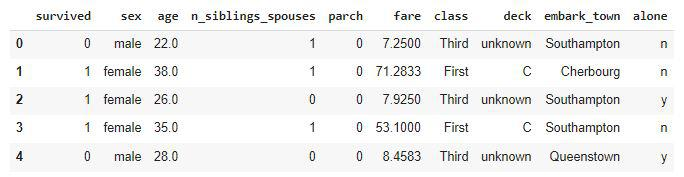Open in App
Not now

# Linear Classifier in Tensorflow

• Last Updated : 21 Nov, 2022

In this article, we will be using tf.estimator.LinearClassifier to build a model and train it on the famous titanic dataset. All of this will be done by using the TensorFlow API.

## Importing Libraries

Python libraries make it easy for us to handle the data and perform typical and complex tasks with a single line of code.

• Pandas – This library helps to load the data frame in a 2D array format and has multiple functions to perform analysis tasks in one go.
• Numpy – Numpy arrays are very fast and can perform large computations in a very short time.
• Matplotlib/Seaborn – This library is used to draw visualizations.

## Python3

 `import` `tensorflow as tf``import` `tensorflow.feature_column as fc`` ` `import` `numpy as np``import` `pandas as pd``import` `matplotlib.pyplot as plt`` ` `import` `warnings``warnings.filterwarnings(``'ignore'``)`

## Importing Dataset

We will import the dataset by using the Tensorflow API for datasets and then load it into the panda’s data frame.

## Python3

 `x_train ``=` `pd.read_csv(``    ``'https://storage.googleapis.com/tf-datasets/titanic/train.csv'``)``x_val ``=` `pd.read_csv(``    ``'https://storage.googleapis.com/tf-datasets/titanic/eval.csv'``)``x_train.head()`

Output:## Python3

 `y_train ``=` `x_train.pop(``'survived'``)``y_val ``=` `x_val.pop(``'survived'``)`

We will need the data for the categorical columns and the numeric(continuous) column present in the dataset separately to initialize our Linear Classifier model.

## Python3

 `objects ``=` `[]``numerics ``=` `[]`` ` `for` `col ``in` `x_train.columns:``    ``if` `x_train[col].dtype ``=``=` `'object'``:``        ``objects.append(col)``         ` `    ``elif` `x_train[col].dtype ``=``=` `'int'``:``        ``objects.append(col)``         ` `    ``else``:``        ``numerics.append(col)`` ` `print``(objects)``print``(numerics)`

Output:

```['sex', 'n_siblings_spouses', 'parch', 'class', 'deck', 'embark_town', 'alone']
['age', 'fare']```

## Python3

 `feat_cols ``=` `[]``for` `feat_name ``in` `objects:``    ``vocabulary ``=` `x_train[feat_name].unique()``    ``feat_cols.append(fc.categorical_column_with_vocabulary_list(feat_name,``                                                                ``vocabulary))`` ` `for` `feat_name ``in` `numerics:``    ``feat_cols.append(fc.numeric_column(feat_name,``                                       ``dtype``=``tf.float32))`

We need to make a callable function that can be passed to the LinearClassifier function.

## Python3

 `def` `make_input_fn(data, label,``                  ``num_epochs``=``10``,``                  ``shuffle``=``True``,``                  ``batch_size``=``32``):``    ``def` `input_function():``        ``ds ``=` `tf.data.Dataset\``            ``.from_tensor_slices((``dict``(data),``                                 ``label))``        ``if` `shuffle:``            ``ds ``=` `ds.shuffle(``1000``)``        ``ds ``=` `ds.batch(batch_size)\``            ``.repeat(num_epochs)``        ``return` `ds``    ``return` `input_function`` ` ` ` `train_input_fn ``=` `make_input_fn(x_train, y_train)``val_input_fn ``=` `make_input_fn(x_val, y_val, num_epochs``=``1``, shuffle``=``False``)`

Now we are good to go to train the tf.estimator.LinearClassifier model using the titanic dataset. Linear Classifier as the name suggests is a Linear model which is used to learn decision boundaries between multiple classes of the object but that should be Linear not non-Linear as we do so in the SVM algorithm.

## Python3

 `linear_est ``=` `tf.estimator.LinearClassifier(feature_columns``=``feat_cols)``linear_est.train(train_input_fn)``result ``=` `linear_est.evaluate(val_input_fn)`` ` `print``(result)`

Output:

```{'accuracy': 0.75,
'accuracy_baseline': 0.625,
'auc': 0.8377411,
'auc_precision_recall': 0.7833674,
'average_loss': 0.47364476,
'label/mean': 0.375, 'loss': 0.4666896,
'precision': 0.6666667,
'prediction/mean': 0.37083066,
'recall': 0.6666667,
'global_step': 200}```

Here we can observe that the model has been evaluated on multiple matrices using the validation dataset and the accuracy obtained is also very satisfactory.

My Personal Notes arrow_drop_up